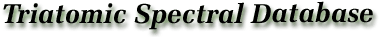### 2. Molecular Parameters and Energy Level Formulation

The treatment of the rotational energy levels and transition frequencies for polyatomic molecules is more complicated than the formulations applied to diatomic molecules . However, since triatomic molecules are the simplest class of polyatomic species, they demonstrate the simplest spectra and interactions that non-rigid polyatomic species are capable of exhibiting. The triatomic molecules can be readily divided into three types as characterized by their spectral properties. These three classes, linear molecules, non-linear molecules, and molecules having doublet (or multiplet) ground electronic states, will be described separately and in that order, since each successive type shows increasingly complex interactions through their rotational spectra.

In spite of the fact that triatomic molecules are the simplest polyatomic species, a thorough discussion of the theoretical background of their rotational spectra and energy level interactions would entail an extensive text in itself, and would require repetition of many excellent treatments readily available. Thus, the discussion which follows deals with the most common cases which will provide the user with the essential definition of quantum numbers, molecular parameters and basic relations employed in the analysis of rotational spectra. For the reader interested in a more detailed description of polyatomic rotational spectral measurements and analysis, we refer to texts on this subject by Townes and Schawlow , Gordy and Cook , Wollrab  and Kroto  which have both detailed and excellent discussions of all facets of rotational spectra. The spectroscopic notation employed follows, as closely as possible, the recommendations of the Joint Commission for Spectroscopy of the International Astronomical Union and the International Union of Pure and Applied Physics .

#### a. Linear Triatomic Molecules

The principal moments of inertia of a linear rigid rotor are along the molecular bond or a-axis for which the inertial moment is zero, and perpendicular to the bond axis in two orthogonal planes through the center-of-mass of the molecule. These are called the b- and c-axis whereby Ic > Ib > Ia determines the labeling of the principal axes. For linear molecules Ib = Ic and Ia = 0, and the rotational constant, B, is related to the moment of the inertia as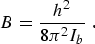(eq1)

The selection rules for rotational transitions of a linear polyatomic molecule are ΔJ = 0, ±1, and Δ=0, ±1, where J is the total angular momentum quantum number excluding nuclear spin and is the vibrational angular momentum quantum number which arises in degenerate bending vibrational states.

Since molecules are not rigid, the effects of molecular vibrations and centrifugal distortion must be included in the model in order to accurately fit the observed rotational spectra. The rotational energy levels are represented as: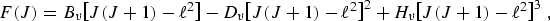(eq2)

where Bυ is the rotational constant for the υth vibrational state, and Dυ and Hυ are the centrifugal distortion constants. The rotational constant can be expressed in terms of its equilibrium value, Be, and rotation-vibration interaction constants, αi, as: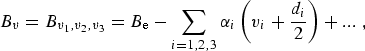(eq3)

neglecting higher order terms. Within this level of approximation rotational transitions from lower state J″ to upper state J′ are expressed: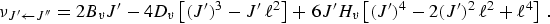(eq4)

The treatment of rotational transitions in excited vibrational states requires additional terms to account for the rotation�vibration interactions. The symmetry species of excited vibrational states are designated as Σ, Π, Δ, etc., when = 0, ± 1, ± 2, etc., respectively. One of the most common rotation-vibration interactions is -type doubling in Π states. In this case each JJ + 1 transition has two components which are indicated as L (lower) and U (upper) components in the tables which follow. The doublet separation is represented as: qυ(υ + 1)(J + 1). In addition ΔJ = 0 transitions are observable with the frequency expressed as: ν = (qυ/2)(υ + 1)J(J + 1). These transitions are also included in the spectral tables. Other rotation-vibration interactions, such as Fermi resonance, often must be included for particular measurements. Since the level of approximation and method of analysis is dependent on the extent and quality of the spectral measurements available, the user should refer to the literature references cited in the tables for details concerning the analysis. For more general treatments of -type doubling and resonance interactions see the texts mentioned earlier [3-6] or the review by D.R. Lide .

Hyperfine structure is observable in a majority of the linear molecules tabulated here. Hyperfine structure stems from nuclear electric quadrupole interaction with the electric field gradient at the nucleus, magnetic interaction of nuclear spin with the field produced by molecular rotation, and interaction between the two nuclear spins. Basically, only the nuclear quadrupole and spin-rotation effects have been observed in microwave rotational spectra, while all of the hyperfine structure interactions of a number of triatomics have been determined from molecular beam electric resonance studies. Since the treatment of these effects can become quite complex and often handled individually for each case, the reader is referred to the literature cited for particular formulations. Rather detailed general treatments of hyperfine structure in molecular spectra can be found in references [3-6] as well as in references to laboratory studies of individual species.

The most common case observed is that for triatomic molecules which contain one nucleus with nuclear spin, I ≥ 1. In this case, the nuclear electric quadrupole and spin�rotation interactions from first order perturbation theory add to the rotational energy via: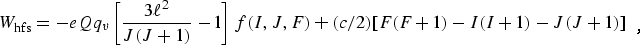(eq5)

where f(I,J,F) is Casimir's function which is tabulated in Appendix I of reference  and Appendix IV of reference . Here F is the total angular momentum quantum number, where F = J + I, J + I - 1, ... |J = I|. The additional selection rule for transitions between rotational levels split by hyperfine structure is ΔF = 0, �1.

When the molecule exhibits hyperfine splittings from more than one nucleus, an exact matrix diagonalization of the Hamiltonian is usually required. Although the vector coupling model to be employed varies according to the magnitude of the interaction between J, I1, and I2, the typical case is to label the nucleus causing the largest interaction as I1, thus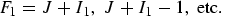(eq6a)
and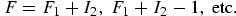(eq6b)

For detailed discussions of the coupling schemes and matrix elements for multiple nuclear electric quadrupole interactions see  and .

#### b. Non-Linear Triatomic Molecules

The majority of polyatomic molecules fall in the asymmetric-top category. When the three principal moments of inertia of a molecule differ, the molecule is classified as an asymmetric top. The energy level formulation for a rigid asymmetric top is considerably more complex than that for symmetric-tops or linear molecules. With the exception of low rotational levels, the rotational energy and transitions cannot be conveniently expressed in simple algebraic terms. Since references [3-6] provide excellent discussions of the usual methods employed in solving the basic rigid asymmetric rotor Hamiltonian: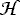2 = A Pa2 + B Pb2 + C Pc2 as well as the more complex Hamiltonian which includes centrifugal distortion=2 +4 +6 + ..., we will not delve into any details of the quantum mechanical formulation, but concentrate on describing the quantum number designations employed in the tables to follow, and provide the basic relationships between the different molecular constant notations used by various authors.

The rotational energy levels are characterized by the three quantum numbers JK-1,K+1 in the King-Hainer-Cross notation. Here, since S=0, J is used rather than N for the rotational angular momentum. When S≠0 we will use NK-1,K+1 to designate the rotational state and J for rotation plus electron spin and orbital angular momenta. The K-1 subscript is the K value in the limiting case of prolate symmetric-top and K+1 corresponds to the limiting case for an oblate symmetric-top. Ray's asymmetry parameter, κ, is often used to characterize the degree of asymmetry:(eq7)

When A ≈ B, κ approaches +1 for the oblate case and when B ≈ C, κ approaches -1 for the prolate case.

#### 1) Selection Rules

In general an asymmetric rotor can exhibit three types of pure rotational transitions if the molecule has non�zero components of the electric dipole moment in the direction of the a, b, and c principal axes. Since triatomic molecules are planar, the dipole moment components can only occur in the a- and b-axis directions. For an asymmetric rotor the selection rules for a-type transitions are: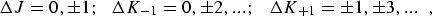(eq8a)

and b-type transitions follow the selection rules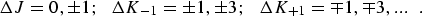(eq8b)

When a triatomic molecule has a symmetry axis, for example in the XY2 molecules, only b-type transitions can occur. In these cases one must also examine the nuclear spin statistics which influence both the selection rules and population of the rotational levels.

#### 2) Rotational and Centrifugal Distortion Constants

Until approximately 1970 the Kivelson and Wilson  formulation of the Hamiltonian for a non-rigid asymmetric rotor was widely employed in analyzing rotational spectra. With the parameter notation employed by Kirchhoff  the Kivelson-Wilson Hamiltonian is: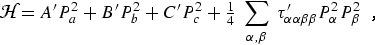(eq9)

where α,β = a,b, or c. For a planar molecule the following planarity relations reduce the six linear combinations of distortion constants to four and provide the determinable parameters shown in column 1 of table 2.1: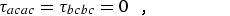(eq10)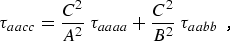(eq11)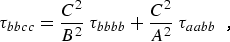(eq12)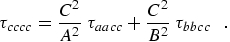(eq13)

For non-planar molecules Dreizler et al. [11,12] found the Kivelson-Wilson distortion constants were indeterminant. Watson [13,14] introduced a new relationship which allows the Kivelson-Wilson Hamiltonian to be expressed in terms of five independent centrifugal distortion coefficients, or linear combination of taus, which eliminates the indeterminancy noted by Dreizler et al. Much of the recent analysis of rotational spectra follow Watson's reformulation [15,16] in the form of a reduced Hamiltonian which simplified the computation of the energy levels.

### Table 2.1. Determinable Rotational and Centrifugal Distortion Constants (P 4)Employed by Various Workers a

Kivelson-Wilson
parameters for
planar molecules
Kirchhoff parameters (following Watson ) Watson
parameters 
A A″ = A′ - 1/2τbbcc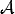B B″ = B′ - 1/2τaacc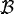C C″ = C′ - 1/2τaabb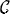τaaaa τaaaa ΔJ
τbbbb τbbbb ΔJK
τabab τcccc ΔK
τaabb τ1 = τaabb′ + τbbcc′ + τccaa δJ
τ2 = (A′ / S) τbbcc′ + (B′ / S) τaacc′ + (C′ / S) τaabb δK
τ3 = [S / (B′ - A′)]τaabb′ + [S / (A′ - C′)]τaacc′ + [S / (C′ - B′)]τbbcc

where

S = A′ + B′ + C

aFor conversion between the various sets of parameters see references , , , and .

Since there is not a unique unitary transformation which allows the nine Kivelson-Wilson parameters to be reduced to eight determinable parameters, several variations of the Watson reduced Hamiltonians are commonly employed in practice. The two most often employed result in the determinable parameters listed in columns 2 and 3 of table 2.1. In reanalyzing the microwave spectra of triatomic molecules, Kirchhoff's  formulation has been used and the planarity conditions have been invoked in the spectral fitting process to fix τ3. See reference  for additional details. The second commonly used formulation is described in detail by Gordy and Cook . Yamada and Winnewisser  have examined the effects of employing different reductions for the three King, Hairier and Cross axis representations Ir, IIr, and IIIr . They provide a useful set of relations between the spectroscopic constants determined in the various reduction procedures and discuss the implications of the τ defect when employing the planarity conditions. When the spectral data requires a higher order Hamiltonian, such as inclusion of P6 terms, generally the first-order perturbation treatment suggested by Watson  has been used. For some light molecules such as H2O and H2S even higher order terms were needed. See Gordy and Cook  or the sources of the spectroscopic constants quoted in the tables on these species.

#### c. Molecules with Doublet Electronic Ground States

The spectra and spectral analysis for molecules with one or more unpaired electrons are substantially more complex, particularly for non-linear free radicals. than for molecules with zero resultant electronic spin. The triatomic species which have doublet, S = 1/2, ground states are HCO, NCO, C2H, CH2, C1O2, HO2, NF2, NH2, and NO2. In addition to the molecular rotational angular momentum, N, the interactions from electronic spin, S, and nuclear spin, I, must be included in the Hamiltonian. Depending on the magnitude of the various interactions, one of the following three coupling schemes are used in limiting cases:

 a) N + S = J; J + I = F   , b) S + I = G; G + N = F   , c) N + I = E; E + S = F   .

These interactions and the Hamiltonian for such molecules are discussed by Lin , Van Vleck , Curl and Kinsey  and others. Curl and Kinsey  have summarized the spectroscopic constant notation employed in the various formulations and developed an alternate method which can be applied to the triatomic species. Since none of these species have been reanalyzed in the present work, the notation employed in the publications cited is followed in the present tables of spectroscopic constants.